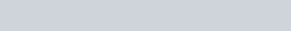# Affinity laws for Rotary pumps

A010. AFFINITY LAWS FOR ROTARY (PD) PUMPS

There are many different types of rotary designs that include gear, vane, lobe, progressive cavity, screw, etc. Rotary pumps are very different from centrifugal pumps:

• They do not have a best efficiency point (BEP).
• There is no impeller shape (specific speed) to consider.
• There is no system curve to match.
• Their capacity is a constant even if the head changes.

Take a look at the following two curves. The one on the left describes a centrifugal pump curve with the curve shape determined by the specific speed number of the impeller. The curve on the right describes the curve we get with a typical rotary pump.H = Head in feet or metersQ = Capacity in gpm, or M3/hr

What happens when you change the speed of each of these type pumps? In the following paragraphs we will look at what happens when you change from 1750 rpm to 3500 rpm. This is a major change in speed, but not all that uncommon.

If you are using a variable speed motor, pulley arrangement or gearbox the speed change might not be as dramatic but the formulas you will be using remain the same.In USCS units (inch units) we will use an example where we will be doubling the speed, but when we do the metric units we will cut the speed in half:

The capacity or amount of fluid you are pumping varies directly with this number.

• Example: 100 gallons per minute x 2 = 200 gallons per minute
• Or in metric: 50 cubic meters per hour x 0,5 = 25 cubic meters per hour

There is no direct change in head with a change in speed. The pump generates whatever head or pressure necessary to pump the capacity.

The horsepower required changes by the number

• Example: A 9 horsepower motor was required to drive the pump at 1750 rpm. How much is required now that you are going to 3500 rpm?
• We would get 9 x 2 = 18 horsepower is now required.
• Or in metric, if a 12 kilowatt motor were required at 3000 rpm. and you decreased the speed to 1500 the new kilowatts required would be 12 x 0,5 = 6,0 kilowatts required for the lower rpm.

The NPSH required varies by the square of the speed

• Example 9 feet x 4 (22) = 36 feet
• Or in metric, 3 meters x 0,25 ( 0,52) = 0,75 meters

Rotary pumps are often used with high viscosity fluids. There is a set of affinity laws for changes in viscosity, but unlike changes in speed the change in viscosity does not give you a direct change in capacity, net positive suction head required (NPSHR), or horsepower.

As an example: an increase in viscosity will increase the capacity because of less slippage, but twice the viscosity does not give you twice the gpm.

Since there are a variety of rotary pump designs operating over a wide range of viscosities, simple statements about changes in operating performance are hard to make, but the following relationships are generally true.

Here are the viscosity affinity laws for rotary pumps:

• Viscosity1>Viscosity= gpm1 > gpm
• Viscosity1>Viscosity2 = BHP1 > BHP2
• Viscosity1>Viscosity2 = NPSHR1 > NPSHR
• Viscosity1>Viscosity2 = No direct affect on differential pressure.

### Posted

• On February 13, 2018